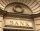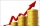# Reducing balance method

A company buys an item having a useful life of 10 years for 1,000,000. If the company depreciates the item by the reducing balance method,
a. Determine the depreciation for the first year.
b. Estimate the depreciation for the second and third years.
c. What is the salvage value of the asset?

a =  100000
b2 =  90000
b3 =  81000
c =  348678.4401

### Step-by-step explanation:Did you find an error or inaccuracy? Feel free to write us. Thank you!Tips to related online calculators
Do you want to convert time units like minutes to seconds?

## Related math problems and questions:

• Car downSarah buys a car costing $12,500. It depreciates value by 8% in the first year, 10% in the second year, and 5% in the third year. Calculate the value of a car after the third year. • Exponential equationDetermine the value of having y in the expression (3^y): (4^-1)=36. Unknown y is a natural number greater than zero. • LoanApply for a$ 59000 loan, the loan repayment period is 8 years, the interest rate 7%. How much should I pay for every month (or every year if paid yearly). Example is for practise geometric progression and/or periodic payment for an annuity.
• Annual interestA loan of 10 000 euro is to be repaid in annual payments over 10 years. Assuming a fixed 10% annual interest rate compounded annually, calculate: (a) the amount of each annual repayment (b) the total interest paid.
• Loan 4Mr. Santos borrowed Php.18,000 at 1.5% interest for one year. What was the total amount he paid for the end of the 1-year term?
• Railways 3Railway Corporation wants to purchase a new machine for $360,000. Management predicts that the machine can produce sales of$220,000 each year for the next 5 years. Expenses are expected to include direct materials, direct labor, and factory overhead (exc
• Suppose 3Suppose that a couple invested Php 50 000 in an account when their child was born, to prepare for the child's college education. If the average interest rate is 4.4% compounded annually, a, Give an exponential model for the situation b, Will the money be
• Volume of woodEvery year, at the same time, an increase in the volume of wood in the forest is measured. The increase is regularly p% compared to the previous year. If in 10 years the volume of wood has increased by 10%, what is the number p?
• PopulationThe town has 65,000 inhabitants. 40 years ago, there were 157,000. How many people will live in a city in 10 years if the population's average rate is as in previous years?
• Profitable companyThree businessman decide to open up their own company. They agree to distribute the yearly profits made in the same ratio as their initial investments. They invest R 50 000, R 75 000 and R25 000, respectively. The profit made by the company in the first y
• You takeYou take out Php 20 000 loan at 5% interest rate. If the interest is compounded annually, a. Give an exponential model for the situation b. How much Will you owe after 10 years?
• PopulationWhat is the population of the city with 3% annual growth, if in 10 years the city will have 60,000 residents?
• Common ratioIf 200 units of a commodity are consumed in a first year, and if the annual rate of increase in consumption is 5% (a) what amount is consumed in the 8th year; (b) in the first 15 years?
• Sum of moneyOn a certain sum of money, invested at the rate of 10% compounded annually, the difference between the interests of the first year and the third year is 315 . Find the sum.
• Annual growthThe population has grown from 25,000 to 33,600 in 10 years. Calculate what was the average annual population growth in%?
• The half lifeThe half-life of a radioactive isotope is the time it takes for a quantity of the isotope to be reduced to half its initial mass. Starting with 145 grams of a radioactive isotope, how much will be left after 3 half-lives?
• Compound interestCompound interest: Clara deposited CZK 100,000 in the bank with an annual interest rate of 1.5%. Both money and interest remain deposited in the bank. How many CZK will be in the bank after 3 years?